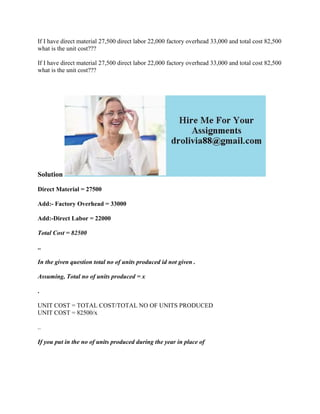Se está descargando tu SlideShare. ×

# If I have direct material 27-500 direct labor 22-000 factory overhead.docx

Anuncio
Anuncio
Anuncio
Anuncio
Anuncio
Anuncio
Anuncio
Anuncio
Anuncio
Anuncio
AnuncioCargando en…3
×

1 de 2 Anuncio

# If I have direct material 27-500 direct labor 22-000 factory overhead.docx

If I have direct material 27,500 direct labor 22,000 factory overhead 33,000 and total cost 82,500 what is the unit cost???

If I have direct material 27,500 direct labor 22,000 factory overhead 33,000 and total cost 82,500 what is the unit cost???

Solution
Direct Material = 27500
Total Cost = 82500
..
In the given question total no of units produced id not given .
Assuming, Total no of units produced = x
.
UNIT COST = TOTAL COST/TOTAL NO OF UNITS PRODUCED
UNIT COST = 82500/x
..
If you put in the no of units produced during the year in place of
.

If I have direct material 27,500 direct labor 22,000 factory overhead 33,000 and total cost 82,500 what is the unit cost???

If I have direct material 27,500 direct labor 22,000 factory overhead 33,000 and total cost 82,500 what is the unit cost???

Solution
Direct Material = 27500
Total Cost = 82500
..
In the given question total no of units produced id not given .
Assuming, Total no of units produced = x
.
UNIT COST = TOTAL COST/TOTAL NO OF UNITS PRODUCED
UNIT COST = 82500/x
..
If you put in the no of units produced during the year in place of
.

Anuncio
Anuncio

### If I have direct material 27-500 direct labor 22-000 factory overhead.docx

1. 1. If I have direct material 27,500 direct labor 22,000 factory overhead 33,000 and total cost 82,500 what is the unit cost??? If I have direct material 27,500 direct labor 22,000 factory overhead 33,000 and total cost 82,500 what is the unit cost??? Solution Direct Material = 27500 Add:- Factory Overhead = 33000 Add:-Direct Labor = 22000 Total Cost = 82500 .. In the given question total no of units produced id not given . Assuming, Total no of units produced = x . UNIT COST = TOTAL COST/TOTAL NO OF UNITS PRODUCED UNIT COST = 82500/x .. If you put in the no of units produced during the year in place of